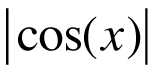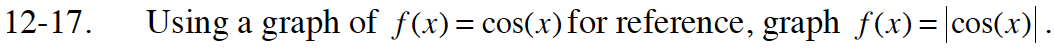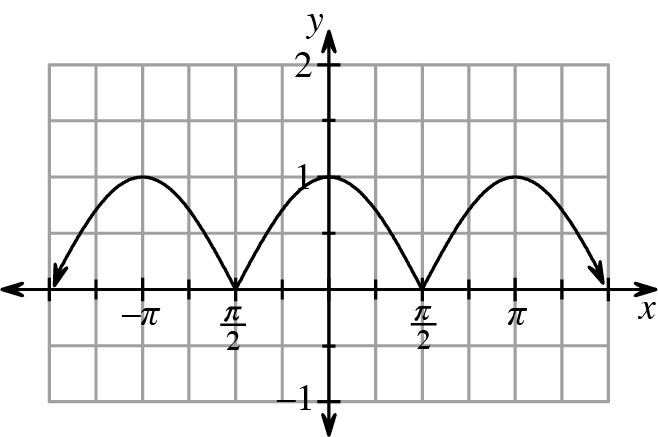Home > CCA2 > Chapter 12 > Lesson 12.1.1 > Problem12-17

12-17.

Using a graph of f(x) = cos(x) for reference, graph f(x) =. Homework Help ✎How does the absolute value change a value?
How would this change look on a graph?